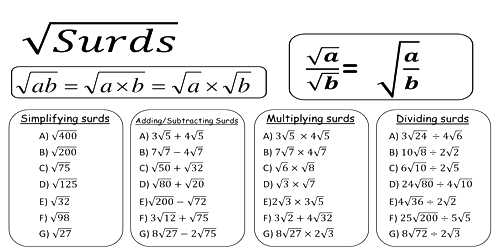Mathematic

# Similar and Dissimilar SurdsSimilar and Dissimilar Surds

A root of a positive real quantity is called a surd if its value cannot be exactly determined. It is a number that can’t be simplified to remove a square root (or cube root etc). For example, each of the quantities √3, ∛7, ∜19, (16)2/5 etc. is a surd.

More Examples:

• √2 (square root of 2) can’t be simplified further so it is a surd
• √4 (square root of 4) CAN be simplified to 2, so it is NOT a surd

Definition of Similar Surds:

Two or more surds are said to be similar or like surds if they have the same surd-factor. or,

Two or more surds are said to be similar or like surds if they can be so reduced as to have the same surd-factor.

For example,

(i) √5, 7√5, 10√5, -3√5, 51/2, 10∙√5, 12∙51/2 are similar surds;

(ii) 7√5, 2√125, 52/5 are similar surds since 2√125 = 2√(5∙5∙5) = 2√5 and 55/2 = √55 = √(5∙5∙5∙5∙5) = 25√5 i.e., each of the given surds can be expressed with the same surd-factor √5.Definition of Dissimilar Surds:

Two or more surds are said to be dissimilar or unlike when they are not similar.

For example, √2, 9√3, 8√5, ∛6, ∜17, 75/6 are unlike surds.

Note: A given rational number can be expressed in the form of a surd of any desired order.

For example, 4 = √16 = ∛64 = ∜256 = n√4n

In general, if a he a rational number then,

x = √x2 = ∛x3 = ∜x4 = n√x2.

Information Source: